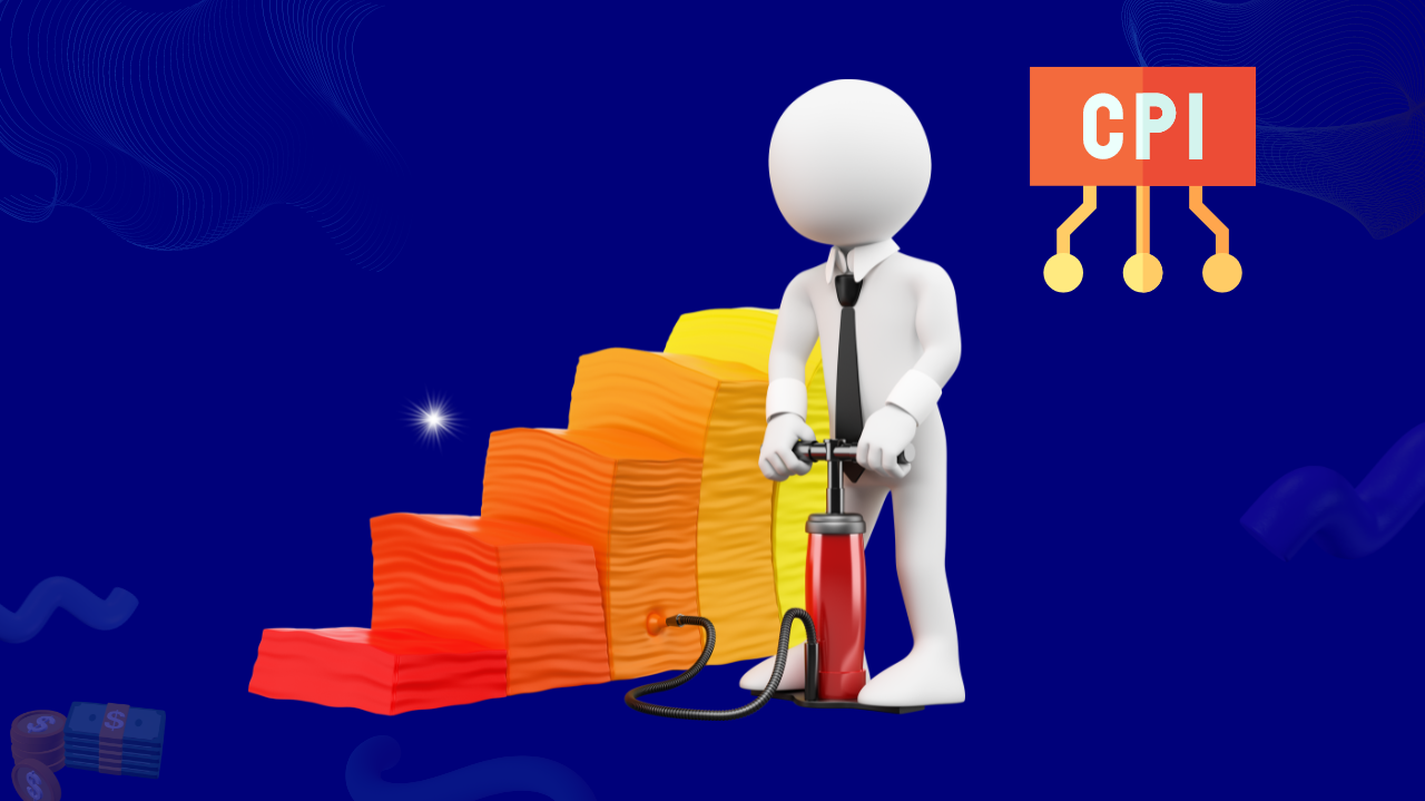How do you calculate the Consumer Price Index?Looking to own 999.9 parts per thousand, LBMA-certified gold? Head over to our website at https://utopianglobal.com/ to invest!

In the current mood of global inflation and possible recessions, it’s important to understand the economic lingo that goes around.

What is the federal funds rate?

What is a trade deficit? What is the Consumer Price Index or CPI?

### What is CPI?

CPI or the Consumer Price Index is a measure of inflation created by the BLS or Bureau of Labor Statistics.

It is used worldwide.

The index was formally created in 1919 but data on food prices started to come through since 1913.

At first, six major groups were used to price the CPI: food, clothing, rent, fuel, house furnishings and miscellaneous.

Over a hundred years, the index has been continuously improved upon in terms of enhanced methodology; updated samples and weights; and expanded coverage.Source : Markus Wrinkler (Pexels)

Today, the CPI is created with 94,000 monthly price quotes collected from 23,000 retail and service establishments.

### How do we calculate CPI?

While we cannot show you how the actual CPI is calculated, this article will use a simple example to show the process behind creating a CPI.

To calculate CPI, you need a base year first.

The base year could be 1918, 1956, 1999 or any year in our calendar.

The base year is simply used as a reference point to calculate how far prices have grown.

For simplicity, the base year’s CPI is locked at 100.

Secondly, you need a basket of goods.

A basket of goods is composed of market items at a set price.

The goods can be everyday items used by consumers such as milk, chicken, bread etc.

For this example, we will use chocolate, coffee and cigarettes for our basket of goods.

Let’s assume the average person would consume 40 packs of cigarettes, 5 boxes of coffee and 25 chocolate bars in a year.

Let’s take the base year as 1982 and fix prices for the following:

• Cigarette pack: \$2 (1982)
• Coffee box: \$5 (1982)
• Chocolate bar: \$1 (1982)

Keep in mind these are base year prices.

Now to calculate CPI, the first step is to add the value of these goods along with their quantities.

We’ll call this a market basket value.

Market Basket Value (1982) = (\$2 x 40) + (\$5 x 5) + (\$1 x 25)

= \$130

So the market value of a basket of goods in 1982 is \$130.

When the actual CPI is calculated, a much larger basket is used, with thousands of goods in higher quantities.

Now to find the CPI for 2022, we need to know the prices for chocolates, cigarettes and coffee in 2022.

Let’s assume that the average person in 2022 consumes the same amount of these goods as they did in 1982 but the prices have increased.

• Cigarette pack: \$3 (2022)
• Coffee box: \$7 (2022)
• Chocolate bar: \$2 (2022)

Let us calculate the market basket value of these goods at 1982 quantities.

Market Basket Value (2022) = (\$3 x 40) + (\$7 x 5) + (\$2 x 25)

= \$205

Now we have the information we need to calculate CPI. Remember the base year’s CPI (1982) is 100.

Here is the formula to calculate 2022’s CPI.

(Market Basket Value in 2022 / Market Basket Value in 1982) x Base year CPI (1982) = CPI in 2022

(\$205/\$130) x 100 = 157.6 ~ 158

So, the CPI for 2022 is 158.

﻿We can calculate inflation from this value as well using another formula.

(158-100)/100 = 58/100 = 0.58 = 58%

We have an inflation rate of 58% which means that over a 40-year period, prices have increased by 58%.

Once you try this method yourself using different goods and quantities, you can understand CPI better.

Why Is the Consumer Price Index Controversial? (investopedia.com)

Consumer Price Index (CPI) Definition (investopedia.com)

Consumer Price Index Frequently Asked Questions : U.S. Bureau of Labor Statistics (bls.gov)

How to calculate the CPI and inflation rate: (uvm.edu)

History : Handbook of Methods: U.S. Bureau of Labor Statistics (bls.gov)

YOU MIGHT ALSO LIKE